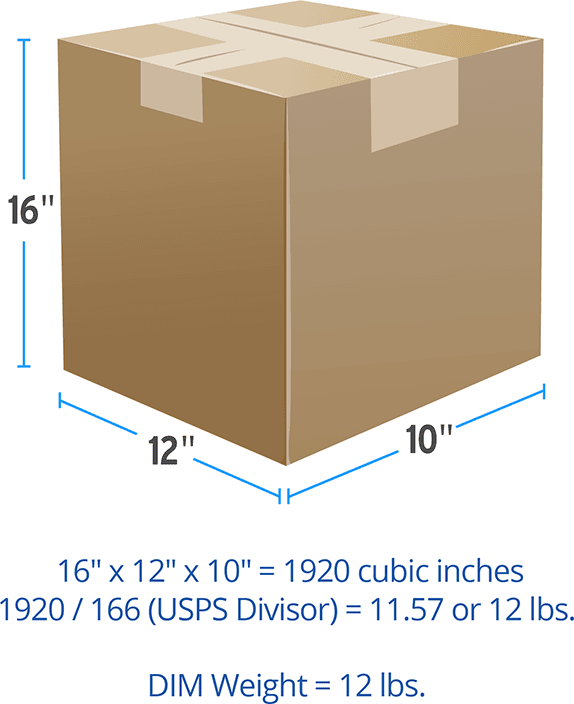## Categories

### How is dimensional weight calculated?

• 2021-07-02 12:15:47
• 1,434

Note: Dimensional weight and it's calculation are function of the US Postal Service and NOT ShipitAPO. But what IS Dimensional Weight?

1. First, you need to determine if your package is greater than one cubic foot (1,728 cubic inches). To do this, use the formula L x W x H. If the result is under 1,728, your package is UNDER one cubic foot. If the result is over 1728, then your package is OVER one cubic foot and DIM Weight pricingmay apply
2. Next, weigh your package to find its actual physical weight.
3. Finally, calculate the DIM Weight by using the USPS Dimensional Weight formula, which is L x W x H/166. (The 166 is known as the DIM Divisor--the USPS uses 166 while other national carriers use 139 as their divisor.) If the result of this calculation yields a higher weight than the actual physical weight of the package, you will pay the DIM Weight price.## Let's look at an example

Supposed you have a package that measures 16" x 12" x 10" and weighs 2 pounds. Use the formula L x W x H to determine if the package is over one cubic foot.

The result is 1,920 cubic inches which is over the 1,728 one cubic foot threshold -- your package will qualify for DIM pricing.

Using the USPS formula 16" L x 12?"W x 10" H/166 we calculate the DIM Weight value to be 11.57, or 12 pounds which is greater than the package actual weight of 2 pounds. Since the DIM Weight of your package is greater than the physical weight, you are required to pay the shipping rate for a 12 pound package. As you can see, using the smallest box size for your products is very beneficial to keep your shipping costs low.